# 什么是机器学习分类算法？【K-近邻算法(KNN)、交叉验证、朴素贝叶斯算法、决策树、随机森林】# 1、K-近邻算法(KNN)

## 1.1 定义

（KNN，K-NearestNeighbor）

## 1.2 距离公式## 1.3 API

• sklearn.neighbors.KNeighborsClassifier(n_neighbors=5,algorithm=’auto’)
• n_neighbors：int,可选（默认= 5），k_neighbors查询默认使用的邻居数
• algorithm：{‘auto’，‘ball_tree’，‘kd_tree’，‘brute’}，可选用于计算最近邻居的算法：‘ball_tree’将会使用 BallTree，‘kd_tree’将使用 KDTree。‘auto’将尝试根据传递给fit方法的值来决定最合适的算法。 (不同实现方式影响效率)
• 其中的你指定的邻居个数实际上是指的当算法计算完一个样本的特征值距离所有其他样本的目标值的距离之后，会根据距离的大小排序，而你的指定的这个参数就是取前多少个值作为判定依据。
• 比如说你指定邻居是5那么如果5个邻居里3个是爱情片，那么可以说这个样本属于爱情片。

## 1.4 案例（预测签到位置）

``````from sklearn.model_selection import train_test_split
from sklearn.neighbors import KNeighborsClassifier
from sklearn.preprocessing import StandardScaler
import pandas as pd

def knncls():
"""
K近邻算法预测入住位置类别
row_id,x,y,accuracy,time,place_id
用户ID,坐标x,坐标y,准确度,时间,位置ID
:return:
"""
# 一、处理数据以及特征工程
# 1、读取收，缩小数据的范围

# 数据逻辑筛选操作 df.query()
data = data.query("x > 1.0 & x < 1.25 & y > 2.5 & y < 2.75")

# 删除time这一列特征
data = data.drop(['time'], axis=1)

print(data)

# 删除入住次数少于三次位置
place_count = data.groupby('place_id').count()

tf = place_count[place_count.row_id > 3].reset_index()

data = data[data['place_id'].isin(tf.place_id)]

# 3、取出特征值和目标值
y = data['place_id']
# y = data[['place_id']]

x = data.drop(['place_id', 'row_id'], axis=1)

# 4、数据分割与特征工程?

# （1）、数据分割
x_train, x_test, y_train, y_test = train_test_split(x, y, test_size=0.3)

# (2)、标准化
std = StandardScaler()

# 队训练集进行标准化操作
x_train = std.fit_transform(x_train)
print(x_train)

# 进行测试集的标准化操作
x_test = std.fit_transform(x_test)

# 二、算法的输入训练预测
# K值：算法传入参数不定的值    理论上：k = 根号(样本数)
# K值：后面会使用参数调优方法，去轮流试出最好的参数[1,3,5,10,20,100,200]
knn = KNeighborsClassifier(n_neighbors=1)

# 调用fit()
knn.fit(x_train, y_train)

# 预测测试数据集，得出准确率
y_predict = knn.predict(x_test)

print("预测测试集类别：", y_predict)

print("准确率为：", knn.score(x_test, y_test))

return None

if __name__ == '__main__':
print()
``````

``````            row_id       x       y  accuracy    place_id
600            600  1.2214  2.7023        17  6683426742
957            957  1.1832  2.6891        58  6683426742
4345          4345  1.1935  2.6550        11  6889790653
4735          4735  1.1452  2.6074        49  6822359752
5580          5580  1.0089  2.7287        19  1527921905
...            ...     ...     ...       ...         ...
29100203  29100203  1.0129  2.6775        12  3312463746
29108443  29108443  1.1474  2.6840        36  3533177779
29109993  29109993  1.0240  2.7238        62  6424972551
29111539  29111539  1.2032  2.6796        87  3533177779
29112154  29112154  1.1070  2.5419       178  4932578245

[17710 rows x 5 columns]
[[-0.39289714 -1.20169649  0.03123826]
[-0.52988735  0.71519711 -0.08049297]
[ 0.84001481  0.82113447 -0.73225846]
...
[-0.64878452 -0.59040929 -0.20153513]
[-1.37250642 -1.33053923 -0.44361946]
[-0.11503962 -1.30477068 -0.22946794]]

Process finished with exit code 0

``````
``````knn.fit(x_train, y_train)

y_predict = knn.predict(x_test)

print("准确率为：", knn.score(x_test, y_test))
``````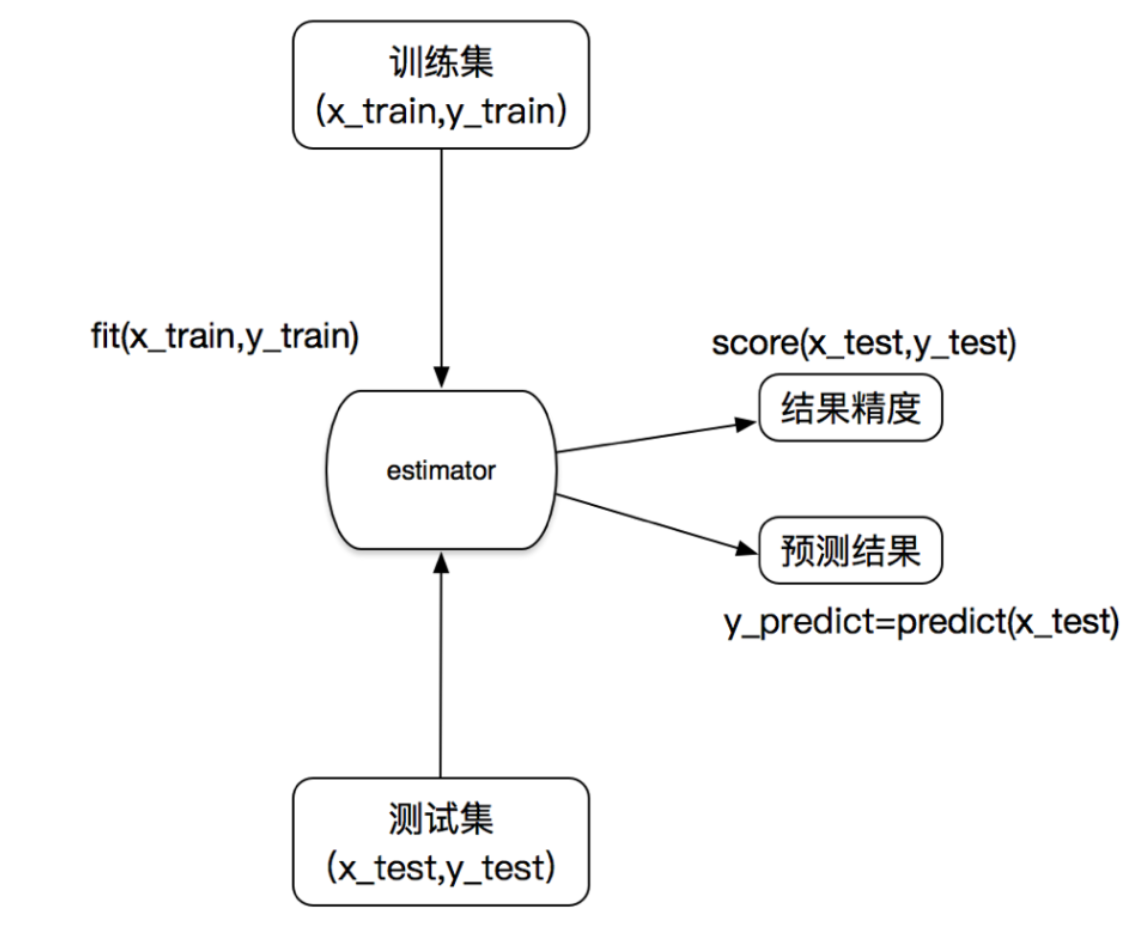### 准确率： 分类算法的评估之一

• 1、k值取多大？有什么影响？

k值取很小：容易受到异常点的影响，比如说有异常的邻居出现在你旁边，那么你的计算结果就会很大程度地受其影响。

k值取很大：受到样本均衡的问题，k值过大相当于选的参考邻居太多了，以至于不知道选哪一个作为标准才好。

• 2、性能问题？

### 小结：

• 优点：
• 简单，易于理解，易于实现，无需训练
• 缺点：
• 懒惰算法，对测试样本分类时的计算量大，内存开销大
• 必须指定K值，K值选择不当则分类精度不能保证
• 使用场景：小数据场景，几千～几万样本，具体场景具体业务去测试

# 2、交叉验证(cross validation)

### 2.1 分析

• 训练集：训练集+验证集
• 测试集：测试集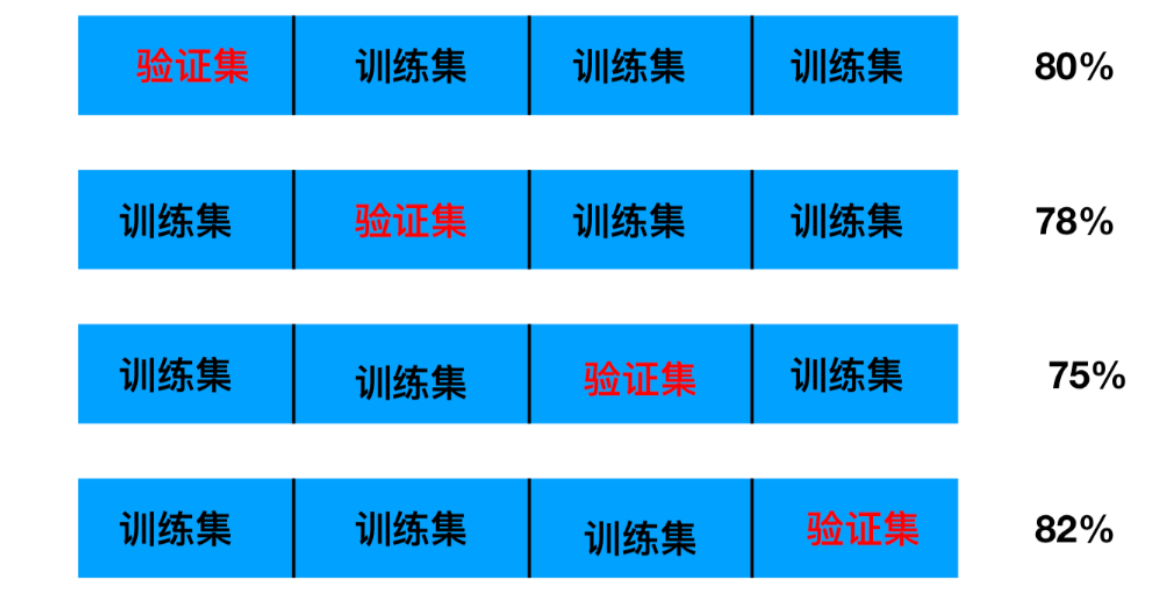• sklearn.model_selection.GridSearchCV(estimator, param_grid=None,cv=None)
• 对估计器的指定参数值进行详尽搜索
• estimator：估计器对象
• param_grid：估计器参数(dict){“n_neighbors”:[1,3,5]}
• cv：指定几折交叉验证
• fit：输入训练数据
• score：准确率
• 结果分析：
• bestscore:在交叉验证中验证的最好结果_
• bestestimator：最好的参数模型
• cvresults:每次交叉验证后的验证集准确率结果和训练集准确率结果

### 2.3 案例（KNN算法—鸢尾花分类）

``````def knn_iris_gscv():
"""
用KNN算法对鸢尾花进行分类，添加网格搜索和交叉验证
:return:
"""
# 1）获取数据

# 2）划分数据集
x_train, x_test, y_train, y_test = train_test_split(iris.data, iris.target, random_state=22)

# 3）特征工程：标准化
transfer = StandardScaler()
x_train = transfer.fit_transform(x_train)
x_test = transfer.transform(x_test)

# 4）KNN算法预估器
estimator = KNeighborsClassifier()

# 加入网格搜索与交叉验证
# 参数准备 n可能的取值 用字典表示   cv = ? 表示几折交叉验证
param_dict = {"n_neighbors": [1, 3, 5, 7, 9, 11]}
estimator = GridSearchCV(estimator, param_grid=param_dict, cv=10)
estimator.fit(x_train, y_train)

# 5）模型评估
# 方法1：直接比对真实值和预测值
y_predict = estimator.predict(x_test)
print("y_predict:\n", y_predict)
print("直接比对真实值和预测值:\n", y_test == y_predict)

# 方法2：计算准确率
score = estimator.score(x_test, y_test)
print("准确率为：\n", score)

# 最佳参数：best_params_
print("最佳参数：\n", estimator.best_params_)
# 最佳结果：best_score_
print("最佳结果：\n", estimator.best_score_)
# 最佳估计器：best_estimator_
print("最佳估计器:\n", estimator.best_estimator_)
# 交叉验证结果：cv_results_
print("交叉验证结果:\n", estimator.cv_results_)

return None
``````

``````y_predict:
[0 2 1 2 1 1 1 2 1 0 2 1 2 2 0 2 1 1 1 1 0 2 0 1 2 0 2 2 2 2 0 0 1 1 1 0 0
0]

[ True  True  True  True  True  True  True  True  True  True  True  True
True  True  True  True  True  True False  True  True  True  True  True
True  True  True  True  True  True  True  True  True  True  True  True
True  True]

0.9736842105263158

{'n_neighbors': 3}

0.9553030303030303

KNeighborsClassifier(n_neighbors=3)

{'mean_fit_time': array([0.00059769, 0.0005955 , 0.00069804, 0.00039876, 0.00049932,
0.00039904]), 'std_fit_time': array([0.00048802, 0.00048625, 0.00063848, 0.00048837, 0.00049932,
0.00048872]), 'mean_score_time': array([0.00144098, 0.00109758, 0.00109758, 0.00089834, 0.00109644,
0.00089748]), 'std_score_time': array([0.00047056, 0.00030139, 0.00029901, 0.0005389 , 0.00029947,
0.00029916]), 'param_n_neighbors': masked_array(data=[1, 3, 5, 7, 9, 11],
mask=[False, False, False, False, False, False],
fill_value='?',
dtype=object), 'params': [{'n_neighbors': 1}, {'n_neighbors': 3}, {'n_neighbors': 5}, {'n_neighbors': 7}, {'n_neighbors': 9}, {'n_neighbors': 11}], 'split0_test_score': array([0.91666667, 0.91666667, 1.        , 1.        , 0.91666667,
0.91666667]), 'split1_test_score': array([1., 1., 1., 1., 1., 1.]), 'split2_test_score': array([0.90909091, 0.90909091, 0.90909091, 0.90909091, 0.90909091,
0.90909091]), 'split3_test_score': array([0.90909091, 1.        , 0.90909091, 0.90909091, 0.90909091,
1.        ]), 'split4_test_score': array([1., 1., 1., 1., 1., 1.]), 'split5_test_score': array([0.90909091, 0.90909091, 0.90909091, 0.90909091, 0.90909091,
0.90909091]), 'split6_test_score': array([0.90909091, 0.90909091, 0.90909091, 1.        , 1.        ,
1.        ]), 'split7_test_score': array([0.90909091, 0.90909091, 0.81818182, 0.81818182, 0.81818182,
0.81818182]), 'split8_test_score': array([1., 1., 1., 1., 1., 1.]), 'split9_test_score': array([1., 1., 1., 1., 1., 1.]), 'mean_test_score': array([0.94621212, 0.95530303, 0.94545455, 0.95454545, 0.94621212,
0.95530303]), 'std_test_score': array([0.04397204, 0.0447483 , 0.06030227, 0.06098367, 0.05988683,
0.0604591 ]), 'rank_test_score': array([4, 1, 6, 3, 4, 1])}

``````

# 3、朴素贝叶斯算法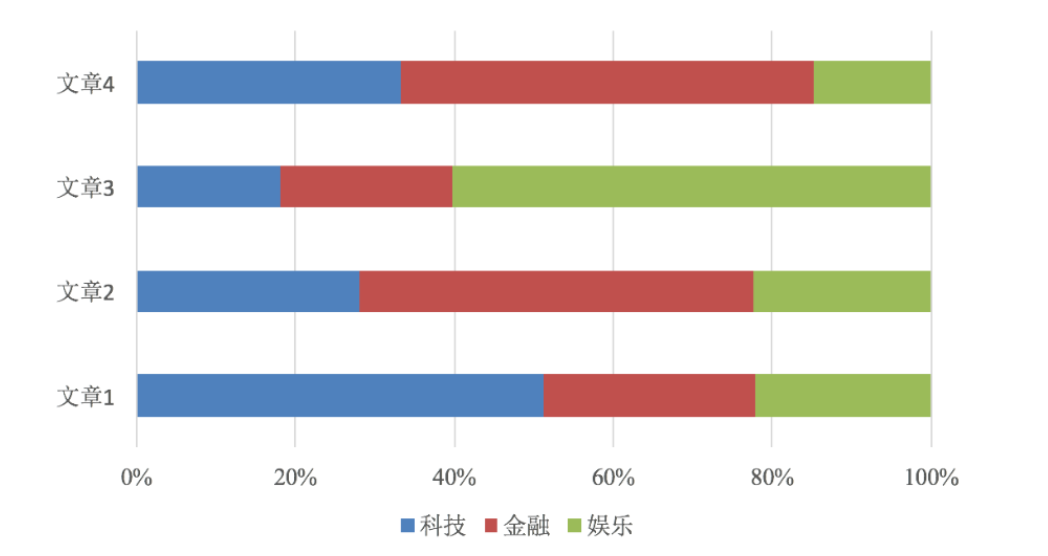## 3.1 贝叶斯公式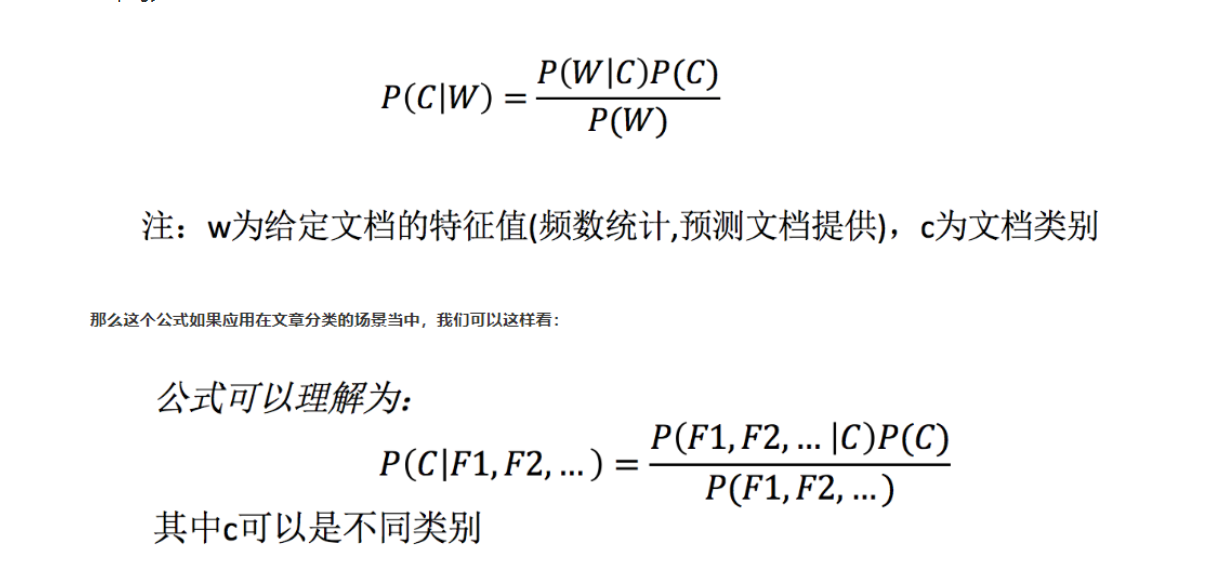• P(C)：每个文档类别的概率(某文档类别数／总文档数量)
• P(W│C)：给定类别下特征（被预测文档中出现的词）的概率
• 计算方法：P(F1│C)=Ni/N （训练文档中去计算）
• Ni为该F1词在C类别所有文档中出现的次数
• N为所属类别C下的文档所有词出现的次数和
• P(F1,F2,…) 预测文档中每个词的概率## 3.2 文档分类计算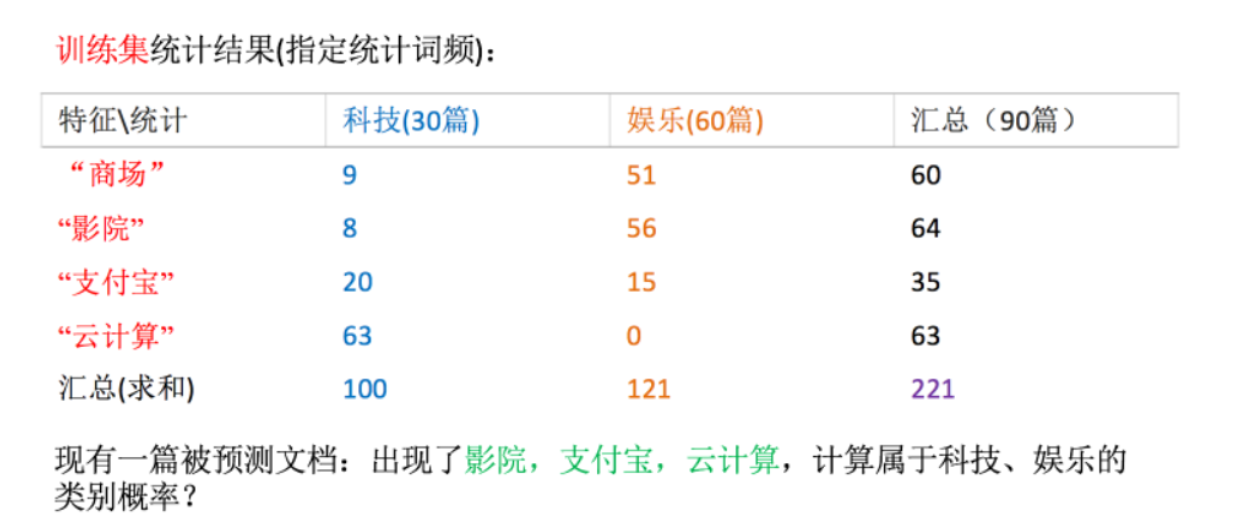``````科技：P(科技|影院,支付宝,云计算) = 𝑃(影院,支付宝,云计算|科技)∗P(科技)=(8/100)∗(20/100)∗(63/100)∗(30/90) = 0.00456109

``````

## 3.3 拉普拉斯平滑系数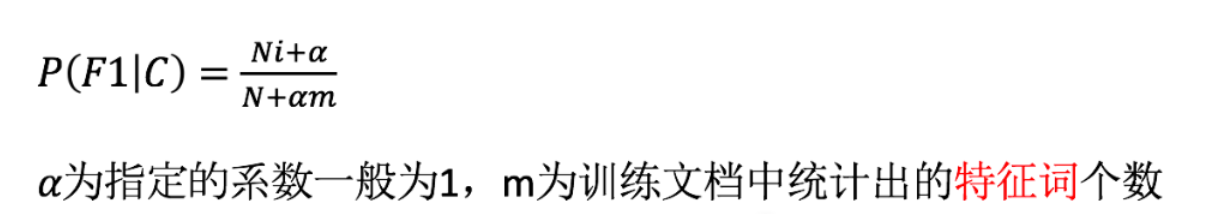``````P(娱乐|影院,支付宝,云计算) =P(影院,支付宝,云计算|娱乐)P(娱乐) =P(影院|娱乐)*P(支付宝|娱乐)*P(云计算|娱乐)P(娱乐)=(56+1/121+4)(15+1/121+4)(0+1/121+1*4)(60/90) = 0.00002
``````

## 3.4 案例（新闻分类）

• sklearn.naive_bayes.MultinomialNB(alpha = 1.0)
• 朴素贝叶斯分类
• alpha：拉普拉斯平滑系数``````def nb_news():
"""
用朴素贝叶斯算法对新闻进行分类
:return:
"""
# 1）获取数据
news = fetch_20newsgroups(subset="all")
print("特征值名字：\n ",news["DESCR"])
# 2）划分数据集
x_train, x_test, y_train, y_test = train_test_split(news.data, news.target)

# 3）特征工程：文本特征抽取-tfidf
transfer = TfidfVectorizer()
x_train = transfer.fit_transform(x_train)
x_test = transfer.transform(x_test)

# 4）朴素贝叶斯算法预估器流程
estimator = MultinomialNB()
estimator.fit(x_train, y_train)

# 5）模型评估
# 方法1：直接比对真实值和预测值
y_predict = estimator.predict(x_test)
print("y_predict:\n", y_predict)
print("直接比对真实值和预测值:\n", y_test == y_predict)

# 方法2：计算准确率
score = estimator.score(x_test, y_test)
print("准确率为：\n", score)

return None

``````

``````y_predict:
[14  1 14 ... 12  6  5]

[ True  True  True ...  True  True False]

0.8423174872665535

``````

### 小结：

• 优点：
• 朴素贝叶斯模型发源于古典数学理论，有稳定的分类效率。
• 对缺失数据不太敏感，算法也比较简单，常用于文本分类。
• 分类准确度高，速度快
• 缺点：
• 由于使用了样本属性独立性的假设，所以如果特征属性有关联时其效果不好

# 4、决策树

## 4.1 认识决策树

### 决策树分类原理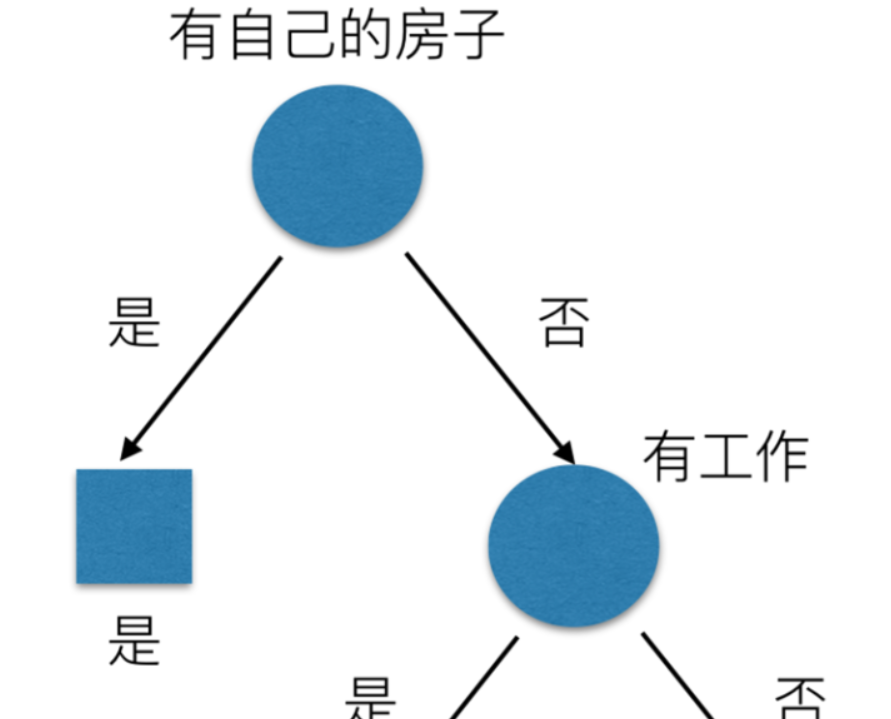## 4.2 信息熵

• H的专业术语称之为信息熵，单位为比特。• 信息和消除不确定性是相联系的

## 4.3 决策树的划分依据之一—信息增益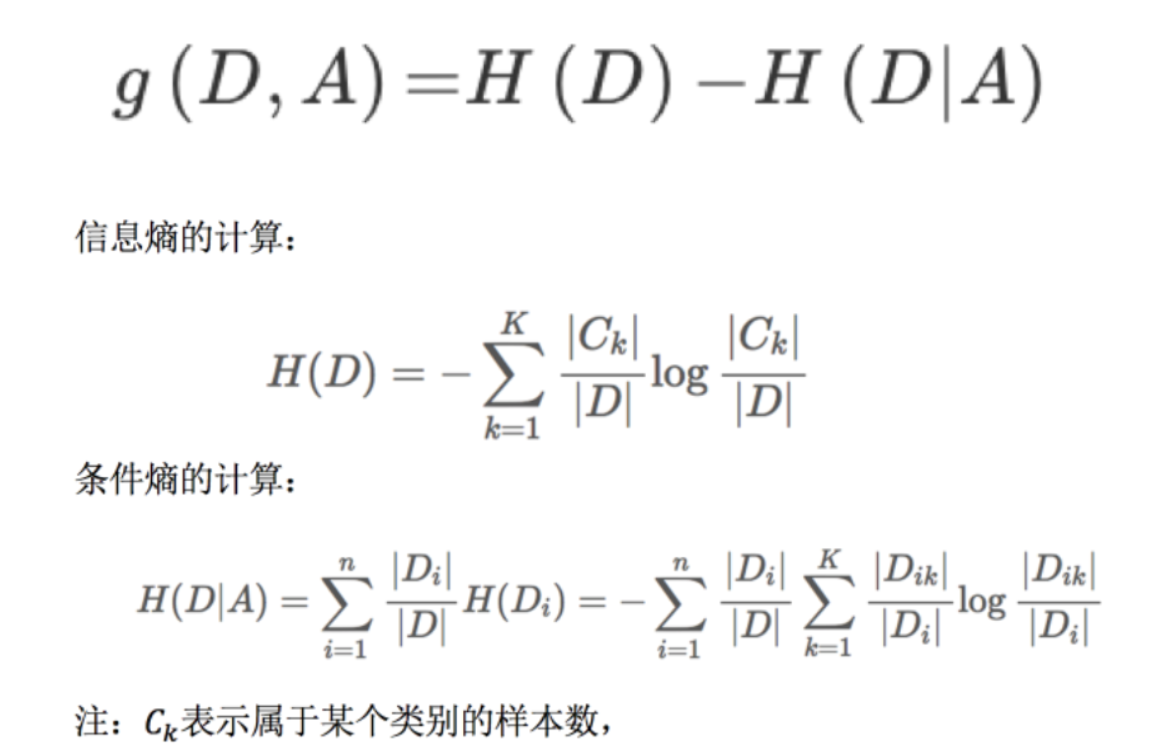``````1、g(D, 年龄) = H(D) -H(D|年龄) = 0.971-[5/15H(青年)+5/15H(中年)+5/15H(老年]

2、H(D) = -(6/15log(6/15)+9/15log(9/15))=0.971

3、H(青年) = -(3/5log(3/5) +2/5log(2/5))
H(中年)=-(3/5log(3/5) +2/5log(2/5))
H(老年)=-(4/5og(4/5)+1/5log(1/5))

``````

## 4.4 决策树的三种算法实现

• ID3
• 信息增益 最大的准则
• C4.5
• 信息增益比 最大的准则
• CART
• 分类树: 基尼系数 最小的准则 在sklearn中可以选择划分的默认原则
• 优势：划分更加细致（从后面例子的树显示来理解）

### 案例（用决策树对鸢尾花进行分类）

• class sklearn.tree.DecisionTreeClassifier(criterion=’gini’, max_depth=None,random_state=None)
• 决策树分类器
• criterion:默认是’gini’系数，也可以选择信息增益的熵’entropy’
• max_depth:树的深度大小
• random_state:随机数种子
• 其中会有些超参数：max_depth:树的深度大小
• 其它超参数我们会结合随机森林讲解
``````def decision_iris():
"""
用决策树对鸢尾花进行分类
:return:
"""
# 1）获取数据集

# 2）划分数据集
x_train, x_test, y_train, y_test = train_test_split(iris.data, iris.target, random_state=22)

# 3）决策树预估器
"""
决策树分类器
criterion:默认是’gini’系数，也可以选择信息增益的熵’entropy’
max_depth:树的深度大小
random_state:随机数种子
"""
estimator = DecisionTreeClassifier(criterion="entropy")
estimator.fit(x_train, y_train)

# 4）模型评估
# 方法1：直接比对真实值和预测值
y_predict = estimator.predict(x_test)
print("y_predict:\n", y_predict)
print("直接比对真实值和预测值:\n", y_test == y_predict)

# 方法2：计算准确率
score = estimator.score(x_test, y_test)
print("准确率为：\n", score)

# 可视化决策树
export_graphviz(estimator, out_file="iris_tree.dot", feature_names=iris.feature_names)

return None
``````

``````y_predict:
[0 2 1 2 1 1 1 1 1 0 2 1 2 2 0 2 1 1 1 1 0 2 0 1 2 0 2 2 2 1 0 0 1 1 1 0 0
0]

[ True  True  True  True  True  True  True False  True  True  True  True
True  True  True  True  True  True False  True  True  True  True  True
True  True  True  True  True False  True  True  True  True  True  True
True  True]

0.9210526315789473
``````

## 小结：

• 优点：
• 简单的理解和解释，树木可视化。
• 缺点：
• 决策树学习者可以创建不能很好地推广数据的过于复杂的树，这被称为过拟合。
• 改进：
• 减枝cart算法(决策树API当中已经实现，随机森林参数调优有相关介绍)
• 随机森林

# 5、随机森林

## 5.2 什么是随机森林

• 例如, 如果你训练了5个树, 其中有4个树的结果是True, 1个数的结果是False, 那么最终投票结果就是True

## 5.3 随机森林原理过程

• 用N来表示训练用例（样本）的个数，M表示特征数目。

• 1、一次随机选出一个样本，重复N次， （有可能出现重复的样本）
• 2、随机去选出m个特征, m <<M，建立决策树
• 采取bootstrap抽样

什么是BootStrap抽样？

• ### bootstrap 独立的

• 为什么要随机抽样训练集？

• 如果不进行随机抽样，每棵树的训练集都一样，那么最终训练出的树分类结果也是完全一样的
• 为什么要有放回地抽样？

• 如果不是有放回的抽样，那么每棵树的训练样本都是不同的，都是没有交集的，这样每棵树都是“有偏的”，都是绝对“片面的”（当然这样说可能不对），也就是说每棵树训练出来都是有很大的差异的；而随机森林最后分类取决于多棵树（弱分类器）的投票表决。

## 5.4 随机森林API

• class sklearn.ensemble.RandomForestClassifier(n_estimators=10, criterion=’gini’, max_depth=None, bootstrap=True, random_state=None, min_samples_split=2)

• 随机森林分类器
• n_estimators：integer，optional（default = 10）森林里的树木数量120,200,300,500,800,1200
• criteria：string，可选（default =“gini”）分割特征的测量方法
• max_depth：integer或None，可选（默认=无）树的最大深度 5,8,15,25,30
• max_features=”auto”,每个决策树的最大特征数量
• If “auto”, then `max_features=sqrt(n_features)`.
• If “sqrt”, then `max_features=sqrt(n_features)` (same as “auto”).
• If “log2”, then `max_features=log2(n_features)`.
• If None, then `max_features=n_features`.
• bootstrap：boolean，optional（default = True）是否在构建树时使用放回抽样
• min_samples_split:节点划分最少样本数
• min_samples_leaf:叶子节点的最小样本数
• 超参数：n_estimator, max_depth, min_samples_split,min_samples_leaf

``````# 随机森林去进行预测
rf = RandomForestClassifier()

param = {"n_estimators": [120,200,300,500,800,1200], "max_depth": [5, 8, 15, 25, 30]}

# 超参数调优
gc = GridSearchCV(rf, param_grid=param, cv=2)

gc.fit(x_train, y_train)

print("随机森林预测的准确率为：", gc.score(x_test, y_test))
``````

## 小结：

• 在当前所有算法中，具有极好的准确率
• 能够有效地运行在大数据集上，处理具有高维特征的输入样本，而且不需要降维
• 能够评估各个特征在分类问题上的重要性

## 几个问题：

### 2、机器学习算法分成哪些类别? 如何分类

1. 监督学习

2. 非监督学习

1. 目标值为离散值就是分类问题

2. 目标值为连续值就是回归问题

### 3、什么是标准化? 和归一化相比有什么优点?

1 total views,  1 views today